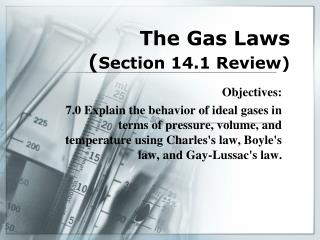DownloadDownload PresentationThe Gas Laws ( Section 14.1 Review)

# The Gas Laws ( Section 14.1 Review)

Télécharger la présentation## The Gas Laws ( Section 14.1 Review)

- - - - - - - - - - - - - - - - - - - - - - - - - - - E N D - - - - - - - - - - - - - - - - - - - - - - - - - - -
##### Presentation Transcript

1. The Gas Laws (Section 14.1 Review) Objectives: 7.0 Explain the behavior of ideal gases in terms of pressure, volume, and temperature using Charles's law, Boyle's law, and Gay-Lussac's law.

2. Boyle’s Lab • Boyle’s law states that the ________ of a gas varies ___________ with _____________. • This means that as volume increase, pressure ______________. • What is the formula for Boyle’s law? • Give an example of application of Boyle’s law.

3. Charles’s Law • How are temperature and volume related? • So as temperature increases, volume __________. • What is the formula for Charles’s law? • What do you have to remember about the temperature in this equation????? • Give an example of application of Charles’s law.

4. Gay-Lussac’s Law • Gay-Lussac’s law says that the ______ of a gas varies _________ with _____________. • So what happens to pressure if temperature increases? • What is the formula for Gay-Lussac’s law? • Again, what units MUST temperature be in? • Give an example of application of Gay-Lussac’s law.

5. Assignment Review • Any questions about Practice Problems? • Any questions about Workbook assignment? • Quiz